PRINTABLE FOR KIDS

XII (12) HSC

XI (11) FYJC
X (10) SSC

### Practice Set 1.2 Linear Equations In Two Variables Class 10th Mathematics Part 1 MHB Solution

Practice Set 1.2

Complete the following table to draw graph of the equations - (I) x+y = 3 (II) x - y =…

(1) x + y = 6; x - y = 4 Solve the following simultaneous equation graphically.…

x + y = 5; x - y = 3 Solve the following simultaneous equation graphically.…

x + y = 0; 2x - y = 9 Solve the following simultaneous equation graphically.…

3x - y = 2; 2x - y = 3 Solve the following simultaneous equation graphically.…

3x - 4y = -7; 5x - 2y = 0 Solve the following simultaneous equation graphically.…

###### Practice Set 1.2

Question 1.

Complete the following table to draw graph of the equations -

(I) x+y = 3 (II) x – y = 4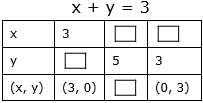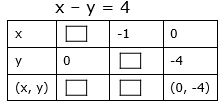(1). In Equation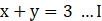i. Put value x=3 in Eq. I we get,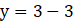⇒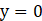ii. Put value y=5 in Eq. I we get,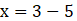⇒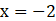iii. Put value y=3 in Eq. I we get,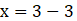⇒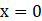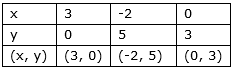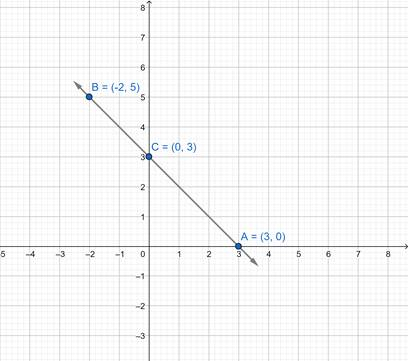(2). In Equation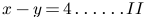i. Put value y=0 in Eq. II we get,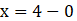⇒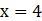ii. Put value x=–1 in Eq. I we get,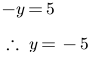iii. Put value y=–4 in Eq. I we get,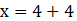⇒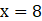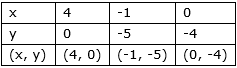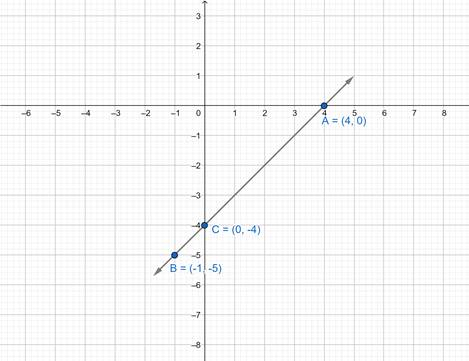Question 2.

Solve the following simultaneous equation graphically.

(1) x + y = 6; x – y = 4

Eq. I =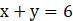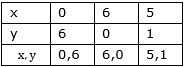Eq. II =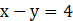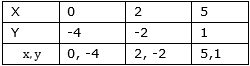Calculating intersecting point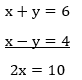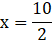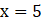Putting x= 5 in Eq.I

5+ y = 6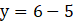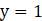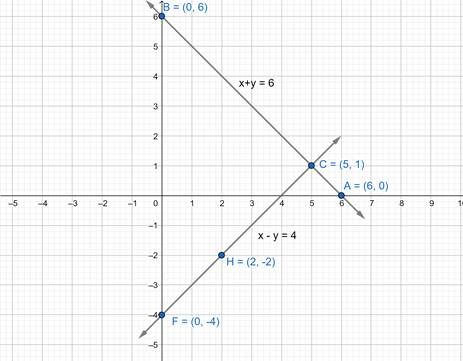Question 3.

Solve the following simultaneous equation graphically.

x + y = 5; x – y = 3

Eq. I =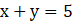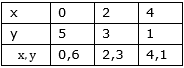Eq. II =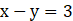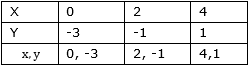Calculating intersecting point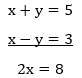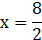Putting x= 4 in Eq.I

4+ y = 5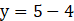Intersection Point (4,1)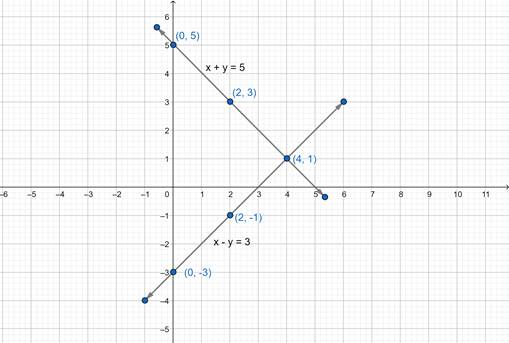Question 4.

Solve the following simultaneous equation graphically.

x + y = 0; 2x – y = 9

Eq. I =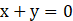Eq. II =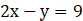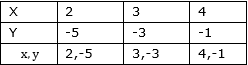Calculating intersecting point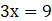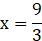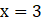Putting x= 3 in Eq.I

3+ y = 0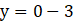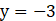Intersection point (3,–3)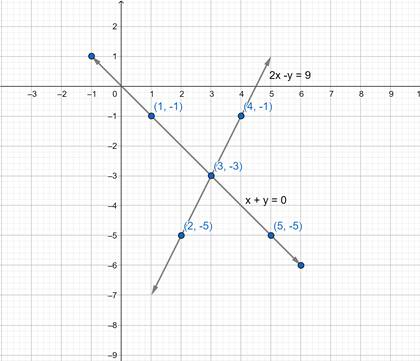Question 5.

Solve the following simultaneous equation graphically.

3x – y = 2; 2x – y = 3

Eq. I =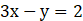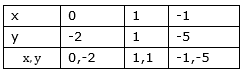Eq. II =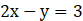Calculating intersecting point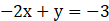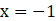Putting x= –1 in Eq.I

3× –1 – y = 2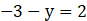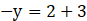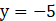Intersection point (–1,–5)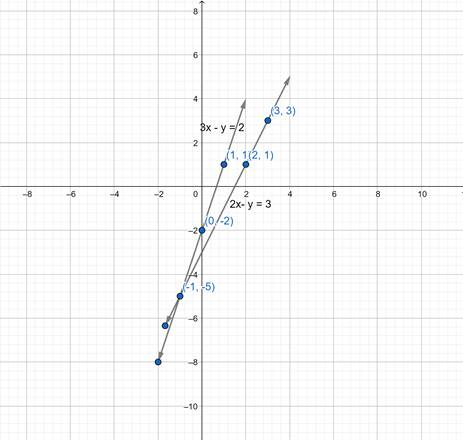Question 6.

Solve the following simultaneous equation graphically.

3x – 4y = –7; 5x – 2y = 0

Eq. I =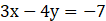When x = 0, 4y = 7, y = 7/4
When y = 0, 3x = -7, x = -7/3

Eq. II =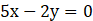When x = 0, y = 0
When x = 1, y = 5/2

Plotting both the graphs we get,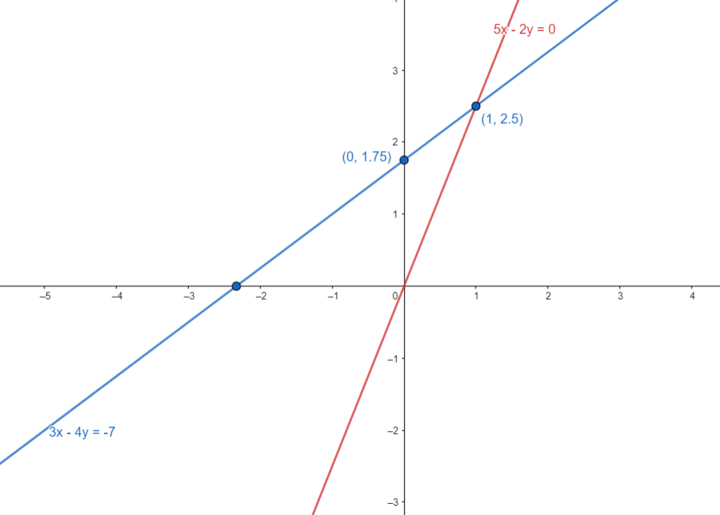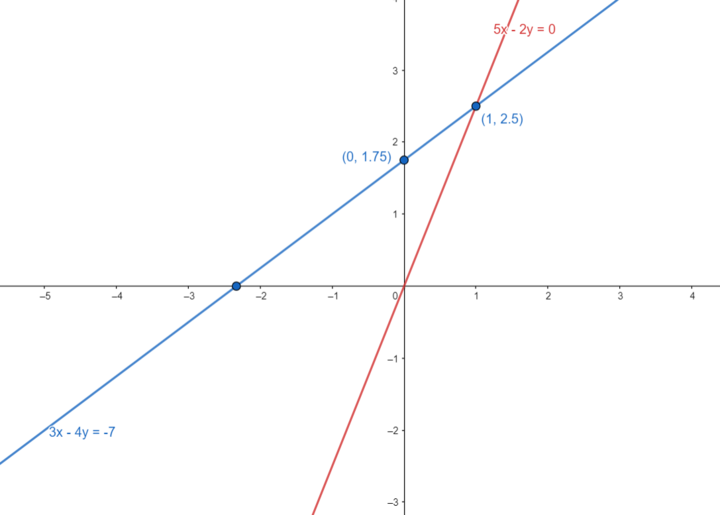Calculating intersecting point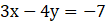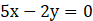Putting x= –1 in Eq.I

3× –1 – y = 2Intersection point (–1,–5)

## PDF FILE TO YOUR EMAIL IMMEDIATELY PURCHASE NOTES & PAPER SOLUTION. @ Rs. 50/- each (GST extra)

SUBJECTS

HINDI ENTIRE PAPER SOLUTION

MARATHI PAPER SOLUTION
SSC MATHS I PAPER SOLUTION
SSC MATHS II PAPER SOLUTION
SSC SCIENCE I PAPER SOLUTION
SSC SCIENCE II PAPER SOLUTION
SSC ENGLISH PAPER SOLUTION
SSC & HSC ENGLISH WRITING SKILL
HSC ACCOUNTS NOTES
HSC OCM NOTES
HSC ECONOMICS NOTES
HSC SECRETARIAL PRACTICE NOTES

2019 Board Paper Solution

HSC ENGLISH SET A 2019 21st February, 2019

HSC ENGLISH SET B 2019 21st February, 2019

HSC ENGLISH SET C 2019 21st February, 2019

HSC ENGLISH SET D 2019 21st February, 2019

SECRETARIAL PRACTICE (S.P) 2019 25th February, 2019

HSC XII PHYSICS 2019 25th February, 2019

CHEMISTRY XII HSC SOLUTION 27th, February, 2019

OCM PAPER SOLUTION 2019 27th, February, 2019

HSC MATHS PAPER SOLUTION COMMERCE, 2nd March, 2019

HSC MATHS PAPER SOLUTION SCIENCE 2nd, March, 2019

SSC ENGLISH STD 10 5TH MARCH, 2019.

HSC XII ACCOUNTS 2019 6th March, 2019

HSC XII BIOLOGY 2019 6TH March, 2019

HSC XII ECONOMICS 9Th March 2019

SSC Maths I March 2019 Solution 10th Standard11th, March, 2019

SSC MATHS II MARCH 2019 SOLUTION 10TH STD.13th March, 2019

SSC SCIENCE I MARCH 2019 SOLUTION 10TH STD. 15th March, 2019.

SSC SCIENCE II MARCH 2019 SOLUTION 10TH STD. 18th March, 2019.

SSC SOCIAL SCIENCE I MARCH 2019 SOLUTION20th March, 2019

SSC SOCIAL SCIENCE II MARCH 2019 SOLUTION, 22nd March, 2019

XII CBSE - BOARD - MARCH - 2019 ENGLISH - QP + SOLUTIONS, 2nd March, 2019

HSC Maharashtra Board Papers 2020

(Std 12th English Medium)

HSC ECONOMICS MARCH 2020

HSC OCM MARCH 2020

HSC ACCOUNTS MARCH 2020

HSC S.P. MARCH 2020

HSC ENGLISH MARCH 2020

HSC HINDI MARCH 2020

HSC MARATHI MARCH 2020

HSC MATHS MARCH 2020

SSC Maharashtra Board Papers 2020

(Std 10th English Medium)

English MARCH 2020

HindI MARCH 2020

Hindi (Composite) MARCH 2020

Marathi MARCH 2020

Mathematics (Paper 1) MARCH 2020

Mathematics (Paper 2) MARCH 2020

Sanskrit MARCH 2020

Important-formula

THANKS